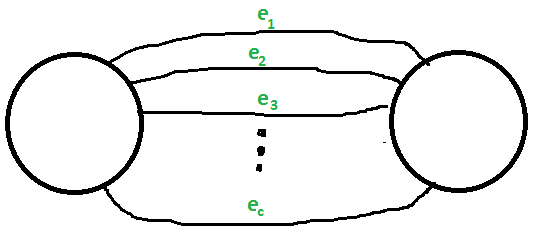# Karger’s algorithm for Minimum Cut | Set 2 (Analysis and Applications)

We have introduced and discussed below Karger’s algorithm in set 1.

```1)  Initialize contracted graph CG as copy of original graph
2)  While there are more than 2 vertices.
a) Pick a random edge (u, v) in the contracted graph.
b) Merge (or contract) u and v into a single vertex (update
the contracted graph).
c) Remove self-loops
3) Return cut represented by two vertices.```

As discussed in the previous post, Karger’s algorithm doesn’t always find min cut. In this post, the probability of finding min-cut is discussed.

Probability that the cut produced by Karger’s Algorithm is Min-Cut is greater than or equal to 1/(n2)

Proof:
Let there be a unique Min-Cut of given graph and let there be C edges in the Min-Cut and the edges be {e1, e2, e3, .. ec}. The Karger’s algorithm would produce this Min-Cut if and only if none of the edges in set {e1, e2, e3, .. ec} is removed in iterations in the main while loop of above algorithm.```c is number of edges in min-cut
m is total number of edges
n is total number of vertices

S1 = Event that one of the edges in {e1, e2,
e3, .. ec} is chosen in 1st iteration.
S2 = Event that one of the edges in {e1, e2,
e3, .. ec} is chosen in 2nd iteration.
S3 = Event that one of the edges in {e1, e2,
e3, .. ec} is chosen in 3rd iteration.

..................
..................

The cut produced by Karger's algorithm would be a min-cut if none of the above
events happen.

So the required probability is P[S1' ∩ S2' ∩ S3' ∩  ............]```

Probability that a min-cut edge is chosen in first iteration:

```Let us calculate  P[S1']
P[S1]  = c/m
P[S1'] = (1 - c/m)

Above value is in terms of m (or edges), let us convert
it in terms of n (or vertices) using below 2 facts..

1) Since size of min-cut is c, degree of all vertices must be greater
than or equal to c.

2) As per Handshaking Lemma, sum of degrees of all vertices = 2m

From above two facts, we can conclude below.
n*c <= 2m
m >= nc/2

P[S1] <= c / (cn/2)
<= 2/n

P[S1] <= c / (cn/2)
<= 2/n

P[S1'] >= (1-2/n) ------------(1)```

Probability that a min-cut edge is chosen in second iteration:

```
P[S1' ∩  S2'] = P[S2' | S1' ] * P[S1']

In the above expression, we know value of P[S1'] >= (1-2/n)

P[S2' | S1'] is conditional probability that is, a min cut is
not chosen in second iteration given that it is not chosen in first iteration

Since there are total (n-1) edges left now and number of cut edges is still c,
we can replace n by n-1 in inequality (1).  So we get.
P[S2' | S1' ] >= (1 - 2/(n-1))

P[S1' ∩  S2'] >= (1-2/n) x (1-2/(n-1))```

Probability that a min-cut edge is chosen in all iterations:

```
P[S1' ∩  S2' ∩ S3'  ∩.......... ∩ Sn-2']

>= [1 - 2/n] * [1 - 2/(n-1)] * [1 - 2/(n-2)] * [1 - 2/(n-3)] *...
... * [1 - 2/(n - (n-4)] * [1 - 2/(n - (n-3)]

>= [(n-2)/n] * [(n-3)/(n-1)] * [(n-4)/(n-2)] * .... 2/4 * 2/3

>= 2/(n * (n-1))
>= 1/n2 ```

How to increase probability of success?
The above probability of success of basic algorithm is very less. For example, for a graph with 10 nodes, the probability of finding the min-cut is greater than or equal to 1/100. The probability can be increased by repeated runs of basic algorithm and return minimum of all cuts found.

Applications:
1) In war situation, a party would be interested in finding minimum number of links that break communication network of enemy.

2) The min-cut problem can be used to study reliability of a network (smallest number of edges that can fail).

3) Study of network optimization (find a maximum flow).

4) Clustering problems (edges like associations rules) Matching problems (an NC algorithm for min-cut in directed graphs would result in an NC algorithm for maximum matching in bipartite graphs)

5) Matching problems (an NC algorithm for min-cut in directed graphs would result in an NC algorithm for maximum matching in bipartite graphs)